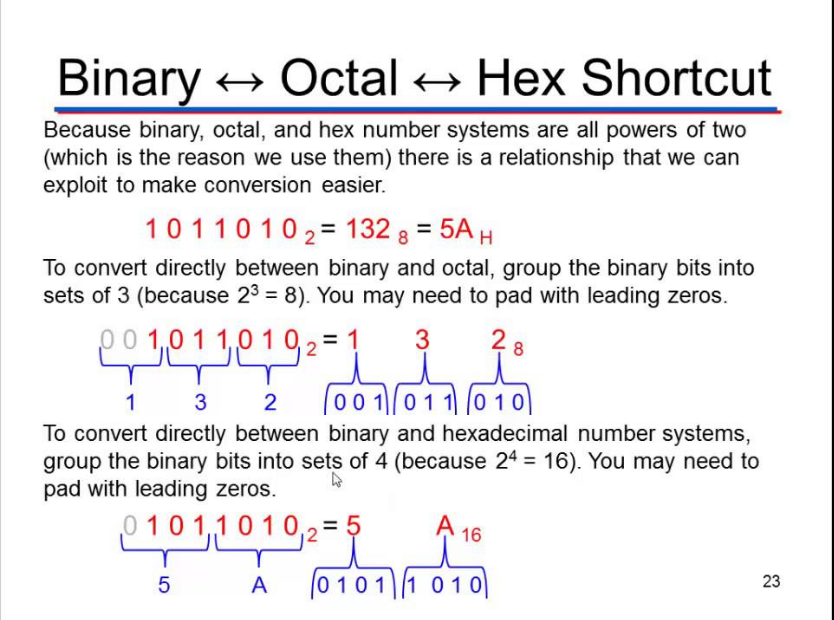# Java Program To Convert Octal To Hexadecimal | Vice Versa

Java program to convert octal to hexadecimal using standard values, recursion, using an array and vice versa ( convert from hexadecimal to octal ( vice versa ) with sample outputs and example programs. The following program has been written in three formats and also vice versa.

In order to get into programming part Just a couple of lines to let you know what and how to calculate octal and hexadecimal in math.

• What is octal?

An octal is a computer-based system with base eight. The digits in math are 0, 1, 2, 3, 4, 5, 6, 7. The value to the base eight represents as single 8 or Zero or 108.

Hexadecimal is base 16 number system.  The hexadecimal is different represent system when compare with the base 10 or octal. The representation of hexadecimal as follows:

0, 1, 2, 3, 4, 5, 6, 7, 8, 9, A, B, C, D, E, F

• How to convert Octal to hex?

A: Here is the procedure: conversion,If you clearly look into the above example you will get to know the method of conversion.

Hope you get it. Here is the complete source code for the above program and vice versa.

## Conversion From Octal To Hexadecimal Java Program

1. Using standard Method

Here we go standard method with sample outputs, as we do already share the conversion you will get to know how this program works. For example, if the number to the base 8 ( octal ) numbers is 16. Than 16 represent as “E” same as followed by 17 represent as ” F “, octal number 18 convert to hexa 10. That’s it

Output:

### 2. Using Recursion

There you go another sample method of using recursion with sample outputs to print octal to hexadecimal.

Output:

#### 3. Convert Octal To Hexadecimal Using Array

Another sample program using array’s: An array is nothing but the continuous allocation of memory. The length of an array is established when an array is created.

Output:

##### 4. Conversion from hexadecimal to octal

Here is the program vice versa: Conversion from hexadecimal to octal with sample outputs and examples.

Output:

x

## Plus Star Pattern Java Program | Patterns

Java program to print plus star pattern program – We have written the below print/draw ...